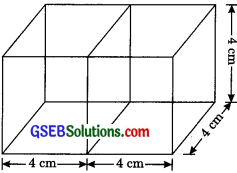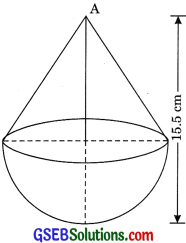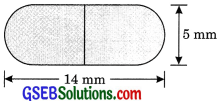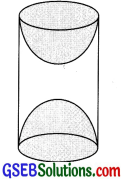# GSEB Solutions Class 10 Maths Chapter 13 Surface Areas and Volumes Ex 13.1

Gujarat Board GSEB Solutions Class 10 Maths Chapter 13 Surface Areas and Volumes Ex 13.1 Textbook Questions and Answers.

## Gujarat Board Textbook Solutions Class 10 Maths Chapter 13 Surface Areas and Volumes Ex 13.1

Question 1.
2 cubes of volume 64 cm3 are joined end to end. Find the surface area of the resulting cuboid.
Solution:
Let the side of the cube by a cm.
Volume of a cube = 64 cm3
a3 = 64
⇒ a = 4 cmBoth cubes combined together form a cuboid of length
l = 4 + 4 = 8 cm
b = 4 cm
h = 4 cm
Surface area of cuboid
= 2[lb + bh + lh]
= 2[ 8 x 4 + 4 x 4 + 8 x 4 ]
= 2 [32 + 16 + 32] = 2 
= 160 cm2Question 2.
A vessel is in the form of a hollow hemisphere mounted by a hollow cylinder. The diameter of the hemisphere is 14 cm and the total height of the vessel is 13 cm. Find the inner surface area of the vessel. (CBSE 2013)
Solution:
Diameter of hemisphere = 14 cmr = 7 cm
Height of cylinder = 13 – 7 = 6 cm
Inner surface area of vessel
= 2πrh + 2πr2
= 2 x $$\frac {22}{7}$$ x (6 + 7)
= 2 x $$\frac {22}{7}$$ x 7(6 + 7)
= 44 x 13 = 572 cm2

Question 3.
A toy is in the form of a cone of radius 3.5 cm mounted on a hemisphere of the same radius. The total height of the toy is 15.5 cm. Find the total surface area of the toy.
Solution:
Herer = 3.5 cm
Height of cone = 15.5 – 3.5 = 12 cm
l = $$\sqrt{r^{2}+h^{2}}$$
= $$\sqrt{3.5^{2}+12^{2}}$$
= $$\sqrt{12.25+144}$$
= $$\sqrt{156.25}$$ = 12.5 cm
Total surface area of toy
= CSA of cone + CSA of hemisphere
= πrl + 2πr2
= πr (l + 2r)
= $$\frac {22}{7}$$ x 3.5 (12.5 + 2 x 3.5)
= $$\frac {22}{7}$$ x 3.5 x 19.5
= 22 x 0.5 x 19.5 = 214.5 cm2Question 4.
A cubical block of side 7 cm is surmounted by a hemisphere. What is the greatest diameter the hemisphere can have? Find the surface area of the solid.
Solution:
Side of cubical block = 7 cm
Diameter of hemisphere = 7 cm
r = $$\frac {7}{2}$$ cmSurface area of solid
= 6a2 + 2πr2 – πr2
= 6a2 + πr2
= 6 x 72 + $$\frac {22}{7}$$ x $$\frac {7}{2}$$ x $$\frac {7}{2}$$
= 6 x 49 + $$\frac {11 x 7}{2}$$
= 294 + 38.5 = 332.5 cm2Question 5.
A hemispherical depression is cut out from one face of a cubical wooden block such that the diameter I of the hemisphere is equal to the edge of the cube. Determine the surface area of the remaining solid.
Solution:
The diameter of cubical wooden block = edge of cubical block = l
Radius of hemispherical depression = $$\frac {l}{2}$$Surface area of block
= 6a2 – πr2 + 2πr2
= 6a2 + πr2
= 6l2 + $$\frac{\pi l^{2}}{4}$$ = l2($$\left(\frac{24+\pi}{4}\right)$$)
= $$\frac {1}{4}$$ l2 (24 + π)

Question 6.
A medicine capsule is in the shape of a cylinder with two hemispheres stuck to each of its ends. The length of the entire capsule is 14 mm and the diameter of the capsule is 5 mm. Find its surface area.Solution:
Diameter of capsule = 5 mm
r = $$\frac {5}{2}$$ mm
Height of cylindrical portion h
= 14 – $$\frac {1}{4}$$ – $$\frac {5}{2}$$ = $$\frac {5}{2}$$ = 14 – 5 = 9 mm
Surface area of capsule
= 2πrh + 2πr2 + 2πr2
= 2πrh + 4πr2
= 2πr(h +2r)
= 2 x $$\frac {22}{7}$$ x $$\frac {5}{2}$$ (9 + 2 + $$\frac {5}{2}$$)
= $$\frac {22}{7}$$ x 5 (9 + 5)
= $$\frac {110}{7}$$ x 14
= 110 x 2 = 220 mm2Question 7.
A tent is in the shape of a cylinder surmounted by a conical top. If the height and diameter of the cylindrical part are 2.1 m and 4m respectively, and the slant height of the top is 2.8 m, find the area of the canvas used for making the tent. Also, find the cost of the canvas of the tent at the rate of 500 per m2. (Note that the base of the tent will not be covered with canvas).
Solution:
Height of cylindrical part h = 2.1 m
Diameter = 4 m
Then r = $$\frac {4}{2}$$
r = 2 mSlant height of conical part l = 2.8 m
Area of canvas = 2πrh + πrl
= πr(2h +l)
= $$\frac {22}{7}$$ x 2 (2 x 2.1 + 2.8)
= $$\frac {44}{7}$$ x (4.2 + 2.8)
= $$\frac {44}{7}$$ x 7 = 44 m2
Total cost of canvas used
= ₹ 500 x 44 = ₹ 22000

Question 8.
From a solid cylinder whose height is 2.4 cm and diameter 1.4 cm, a conical cavity of the same height and same diameter is hollowed out. Find the total surface area of the remaining solid to the nearest cm2.
Solution:
Height = 2.4 m
and diameter = 1.4 cm
r = 0.7 cm
and radius of conical cavity or base
= 0.7 cmHeight of the solid cylinder = 2.4 cm
Height of conical cylinder = 2.4 cm
l = $$\sqrt{r^{2}+h^{2}}$$
= $$\sqrt{(0.7)^{2}+(2.4)^{2}}$$
= $$\sqrt{0.49+5.76}$$
= $$\sqrt{6.25}$$
l = 2.5cm
Total surface area of remaining solid
= 2πrh + πr2 + πrl
= 2 x $$\frac {22}{7}$$ x o.7 x 2.4 + $$\frac {22}{7}$$ x (0.7)2 x $$\frac {22}{7}$$ x 0.7 x 2.5
= 44 x 0.1 x 2.4 + 22 x 0.1 x 0.7 + 22 x 0.1 x 2.5
= 10.56 + 1.54 + 5.5
= 17.6 cm2 – 18 cm2 (nearest cm2)Question 9.
A wooden article was made by scooping out a hemisphere from each end of a solid cylinder, as shown in the figure. If the height of the cylinder is 10 cm, and its base is of radius 3.5 cm, find the total surface area of the article.
Solution:
Here:h = 10cm
r = 3.5 cm
Total surface area of article
= 2πrh + 2πr2 + 2πr2
= 2πrh + 4πr2 = 2πr (h + 2r)
= 2 x $$\frac {22}{7}$$ x 3.5 (10 + 2 x 3.5)
= 44 x 0.5 (10 + 7)
= 22x 17 = 374 cm2